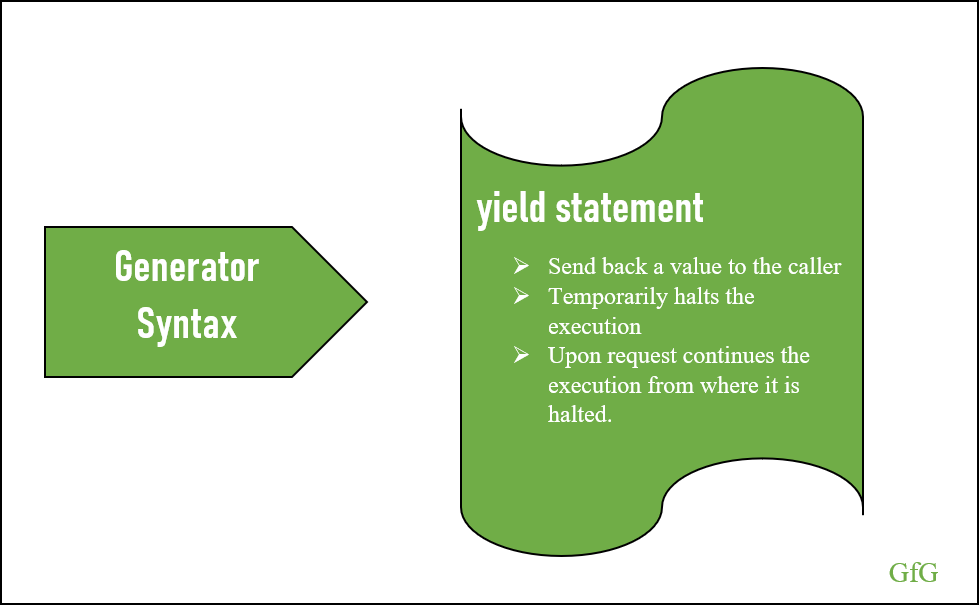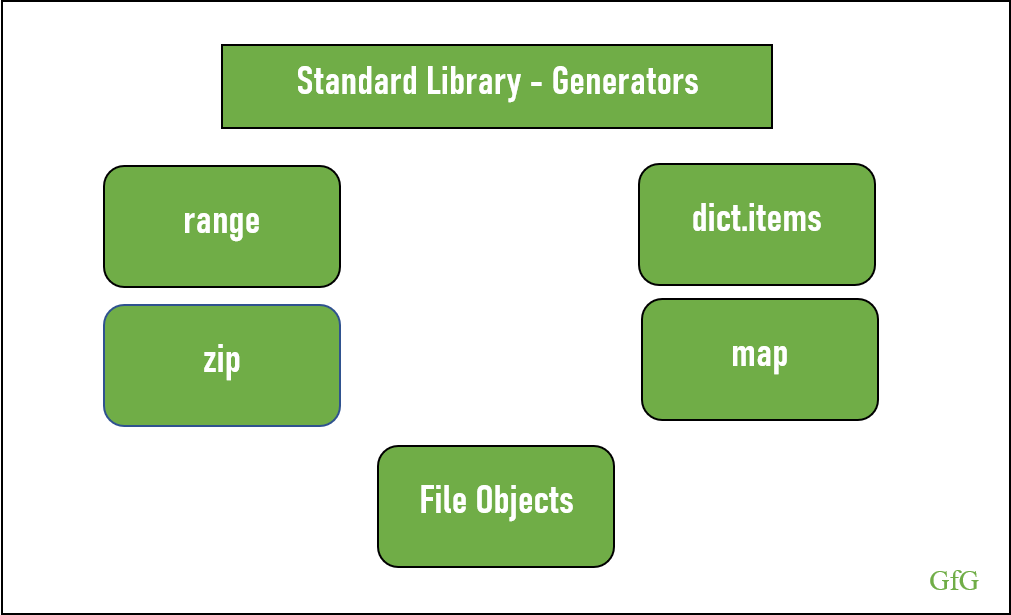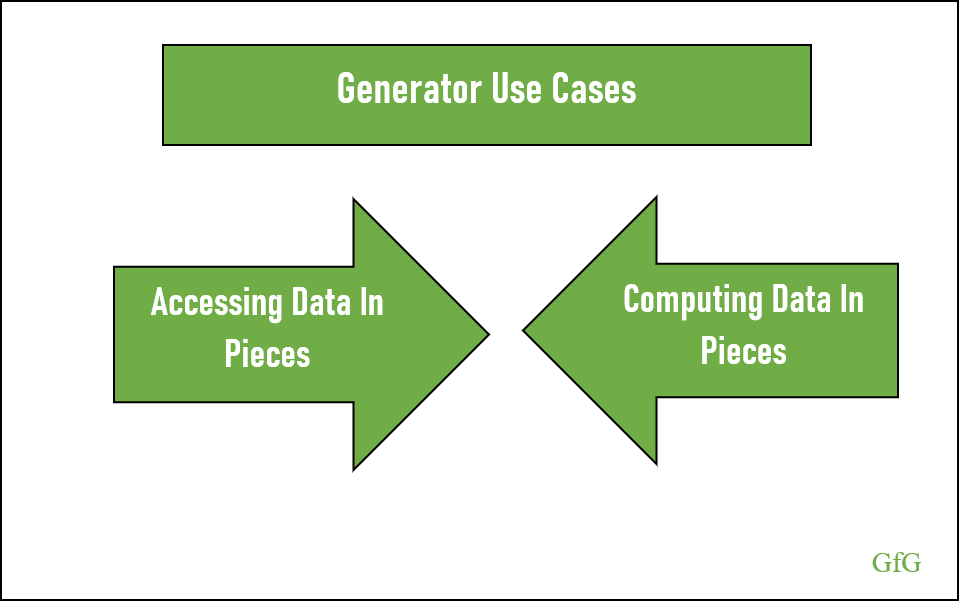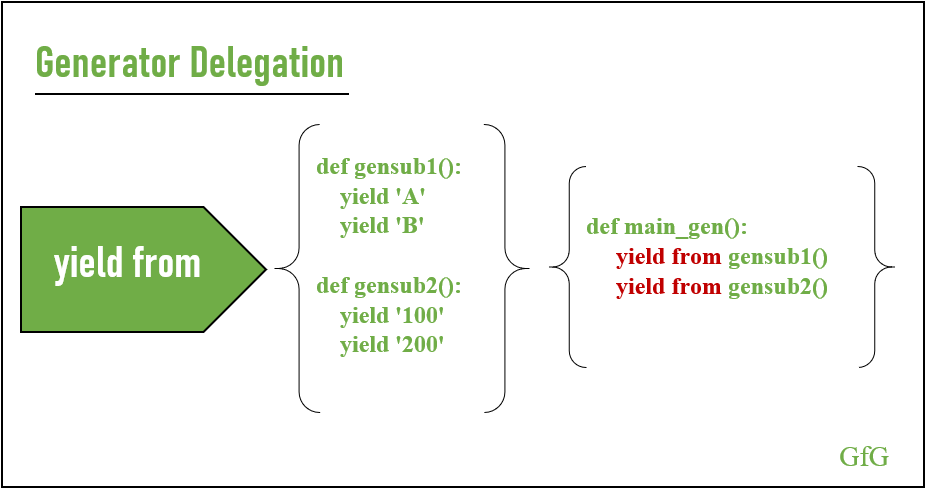Using Generators for substantial memory savings in Python

• Last Updated : 10 May, 2020

When memory management and maintaining state between the value generated become a tough job for programmers, Python implemented a friendly solution called Generators.Generators

With Generators, functions evolve to access and compute data in pieces. Hence functions can return the result to its caller upon request and can maintain its state. Generators maintain the function state by halting the code after producing the value to the caller and upon request, it continues execution from where it is left off.
Since Generator access and compute value on-demand, a large chunk of data doesn’t need to be saved in memory entirely and results in substantial memory savings.

Generator Syntaxyield statement

We can say that a function is a generator when it has a yield statement within the code. Like in a return statement, the yield statement also sends a value to the caller, but it doesn’t exit the function’s execution. Instead, it halts the execution until the next request is received. Upon request, the generator continues executing from where it is left off.

 def primeFunction():    prime = None    num = 1    while True:        num = num + 1          for i in range(2, num):             if(num % i) == 0:                prime = False                break            else:                prime = True          if prime:                          # yields the value to the caller             # and halts the execution            yield num  def main():          # returns the generator object.    prime = primeFunction()          # generator executes upon request    for i in prime:        print(i)        if i > 50:            break  if __name__ == "__main__":    main()

Output

3
5
7
11
13
17
19
23
29
31
37
41
43
47
53

Communication With Generatornext, stopIteration and send

How the caller and generator communicate with each other? Here we will discuss 3 in-built functions in python. They are:

• next
• stopIteration
• send

next

The next function can request a generator for its next value. Upon request, the generator code executes and the yield statement provides the value to the caller. At this point, the generator halts the execution and waits for the next request. Let’s dig deeper by considering a Fibonacci function.

 def fibonacci():    values = []    while True:          if len(values) < 2:            values.append(1)        else :                          # sum up the values and             # append the result            values.append(sum(values))                          # pop the first value in             # the list            values.pop(0)          # yield the latest value to         # the caller        yield values[-1]        continue  def main():    fib = fibonacci()    print(next(fib))  # 1    print(next(fib))  # 1    print(next(fib))  # 2    print(next(fib))  # 3    print(next(fib))  # 5  if __name__ == "__main__":    main()

Output

1
1
2
3
5
• Creating the generator object by calling the fibonacci function and saving its returned value to fib. In this case, the code hasn’t run, the python interpreter recognizes the generator and returns the generator object. Since the function has a yield statement the generator object is returned instead of a value.
 fib = fibonacci()fib

Output

generator object fibonacci at 0x00000157F8AA87C8
• Using next function, the caller requests a value to the generator and the execution begins.

next(gen)
Output

1
• Since the values list is empty the code within the ‘if statement’ is executed and `1` is appended to the values list. Next, the value is yielded to the caller using yield statement and the execution halts. The point to note here is that the execution halts before executing the continue statement.
 # values = []if len(values) < 2:      # values =     values.append(1)  # 1yield values[-1]continue
• Upon the second request the code continues execution from where it left off. Here it executes from the `continue` statement and passes the control to the while loop.
Now the values list contains a value from the first request. Since the length of the ‘values` is 1 and is less than 2 the code within the ‘if statement’ executes.
 # values = if len(values) < 2:      # values = [1, 1]    values.append(1)  # 1 (latest value is provided # to the caller)yield values[-1]continue
• Again, the value is requested using next(fib) and the execution starts from `continue` statement. Now the length of the values is not less than 2. Hence it enters the else statement and sums up the values in the list and appends the result. The pop statement removes the first element from the list and yields the latest result.
 # values = [1, 1]else:          # values = [1, 1, 2]    values.append(sum(values))          # values = [1, 2]    values.pop(0)      # 2yield values[-1]continue
• Your request for more values will repeat the pattern and yield the latest value

StopIteration

StopIteration is a built-in exception that is used to exit from a Generator. When the generator’s iteration is complete, it signals the caller by raising the StopIteration exception and it exits.

Below code explains the scenario.

 def stopIteration():    num = 5    for i in range(1, num):        yield i  def main():    f = stopIteration()          # 1 is generated    print(next(f))          # 2 is generated    print(next(f))          # 3 is generated    print(next(f))          # 4 is generated    print(next(f))          # 5th element - raises    # StopIteration Exception    next(f)  if __name__ == "__main__":    main()

Output

1
2
3
4
Traceback (most recent call last):
File “C:\Users\Sonu George\Documents\GeeksforGeeks\Python Pro\Generators\stopIteration.py”, line 19, in
main()
File “C:\Users\Sonu George\Documents\GeeksforGeeks\Python Pro\Generators\stopIteration.py”, line 15, in main
next(f) # 5th element – raises StopIteration Exception
StopIteration

The below code explains another scenario, where a programmer can raise StopIteration and exit from the generator.
raise StopIteration

 def stopIteration():    num = 5    for i in range(1, num):        if i == 3:            raise StopIteration        yield i            def main():    f = stopIteration()          # 1 is generated    print(next(f))          # 2 is generated    print(next(f))          # StopIteration raises and     # code exits    print(next(f))    print(next(f))            if __name__ == "__main__":    main()

Output

1
2
Traceback (most recent call last):
File “C:\Users\Sonu George\Documents\GeeksforGeeks\Python Pro\Generators\stopIteration.py”, line 5, in stopIteration
raise StopIteration
StopIteration

The above exception was the direct cause of the following exception:

Traceback (most recent call last):
File “C:\Users\Sonu George\Documents\GeeksforGeeks\Python Pro\Generators\stopIteration.py”, line 19, in
main()
File “C:\Users\Sonu George\Documents\GeeksforGeeks\Python Pro\Generators\stopIteration.py”, line 13, in main
print(next(f)) # StopIteration raises and code exits
RuntimeError: generator raised StopIteration

send

So far, we have seen how generator yield values to the invoking code where the communication is unidirectional. As of now, the generator hasn’t received any data from the caller.
In this section, we will discuss the `send` method that allows the caller to communicate with the generator.

 def factorial():    num = 1    while True:        factorial = 1          for i in range(1, num + 1):                          # determines the factorial            factorial = factorial * i                      # produce the factorial to the caller        response = yield factorial          # if the response has value        if response:                          # assigns the response to             # num variable            num = int(response)        else:                          # num variable is incremented            # by 1            num = num + 1  def main():    fact = factorial()    print(next(fact))    print(next(fact))    print(next(fact))    print(fact.send(5))   # send    print(next(fact))  if __name__ == "__main__":    main()

Output

1
2
6
120
720

The generator yields the first three values (1, 2 and 6) based on the request by the caller (using the next method) and the fourth value (120) is produced based on the data (5) provided by the caller (using send method).
Let’s consider the 3rd data (6) yielded by the generator. Factorial of 3 = 3*2*1, which is yielded by the generator and the execution halts.

factorial = factorial * i

At this point, the caller uses the `send` method and provide the data ‘5`. Hence generator executes from where it is left off i.e. saves the data sent by the caller to the `response` variable (response = yield factorial). Since the `response` contains a value, the code enters the `if` condition and assigns the response to the `num` variable.

 if response:    num = int(response)

Now the flow passes to the `while` loop and determines the factorial and is yielded to the caller. Again, the generator halts the execution until the next request.

If we look into the output, we can see that the order got interrupted after the caller uses the `send` method. More precisely, within the first 3 requests the output as follows:
Factorial of 1 = 1
Factorial of 2 = 2
Factorial of 3 = 6

But when the user sends the value 5 the output becomes 120 and the `num` maintains the value 5. On the next request (using `next`) we expect num to get incremented based on last `next` request (i.e. 3+1 = 4) rather than the `send` method. But in this case, the `num` increments to 6 (based on last value using `send`) and produces the output 720.

The below code shows a different approach in handling values sent by the caller.

 def factorial():    num = 0    value = None    response = None    while True:        factorial = 1               if response:            value = int(response)        else:            num = num + 1            value = num                  for i in range(1, value + 1):            factorial = factorial * i        response = yield factorial           def main():    fact = factorial()    print(next(fact))    print(next(fact))    print(next(fact))    print(fact.send(5))   # send    print(next(fact))  if __name__ == "__main__":    main()

Output

1
2
6
120
24

Standard Library – GeneratorsStandard Library

• range
• dict.items
• zip
• map
• File Objects

range

Range function returns an iterable range object and its iterator is a generator. It returns the sequential value which starts from the lower limit and continues till the upper limit is reached.

 def range_func():    r = range(0, 4)    return r  def main():    r = range_func()    iterator = iter(r)    print(next(iterator))    print(next(iterator))  if __name__ == "__main__":    main()

Output

0
1

dict.items

Dictionary class in python provides three iterable methods to iterate the dictionary. They are key, values and items and their iterators are generators.

 def dict_func():    dictionary = {'UserName': 'abc', 'Password':'a@123'}    return dictionary  def main():    d = dict_func()    iterator = iter(d.items())    print(next(iterator))    print(next(iterator))  if __name__ == "__main__":    main()

Output

zip

zip is a built-in python function which takes multiple iterable object and iterates all at once. They yield the first element from each iterable, then the second and so on.

 def zip_func():    z = zip(['a', 'b', 'c', 'd'], [1, 2, 3, 4])    return z  def main():    z = zip_func()    print(next(z))    print(next(z))    print(next(z))  if __name__ == "__main__":    main()

Output

('a', 1)
('b', 2)
('c', 3)

map

The map function takes function and iterables as parameters and computes the result of the function to each item of the iterable.

 def map_func():    m = map(lambda x, y: max([x, y]), [8, 2, 9], [5, 3, 7])    return m  def main():    m = map_func()    print(next(m))  # 8 (maximum value among 8 and 5)    print(next(m))  # 3 (maximum value among 2 and 3)    print(next(m))  # 9 (maximum value among 9 and 7)  if __name__ == "__main__":    main()

Output

8
3
9

File Object

Even though the file object has a readline method to read the file line by line, it supports the generator pattern. One difference is that here the readline method catches the StopIteration exception and returns an empty string once the end of file is reached, which is different while using the next method.

While using next method, file object yields the entire line including the newline (\n) character

 def file_func():    f = open('sample.txt')    return f  def main():    f = file_func()    print(next(f))    print(next(f))      if __name__ == "__main__":    main()

Input: sample.txt

Rule 1
Rule 2
Rule 3
Rule 4

Output

Rule 1

Rule 2

Generators Use CaseGenerator Use Cases

The Fundamental concept of Generator is determining the value on demand. Below we will discuss two use cases that derive from the above concept.

• Accessing Data in Pieces
• Computing Data in Pieces

Accessing Data in Pieces

Why do we need to access data in pieces? The question is valid when the programmer has to deal with a large amount of data, say reading a file and so. In this case, making a copy of data and processing it is not a feasible solution. By using generators, programmers can access the data one at a time. When considering file operation, the user can access data line by line and in case of a dictionary, two-tuple at a time.
Hence Generator is an essential tool to deal with a large chunk of data that avoids unnecessary storage of the data and results in substantial memory savings.

Computing Data in Pieces

Another reason to write a Generator is its ability to compute data on request. From the above Fibonacci function, one can understand that the generator produces the value on demand. This process avoids unnecessary computing and storing the values and hence can increase the performance and also results in substantial memory savings.
Another point to note is that the generator’s ability to compute an infinite number of data.

Generator Delegationyield from

The generator can invoke another generator as a function does. Using ‘yield from’ statement a generator can achieve this, and the process is called Generator Delegation.
Since the generator is delegating to another generator, the values sent to the wrapping generator will be available to the current delegate generator.

 def gensub1():    yield 'A'    yield 'B'  def gensub2():    yield '100'    yield '200'  def main_gen():    yield from gensub1()    yield from gensub2()      def main():    delg = main_gen()    print(next(delg))    print(next(delg))    print(next(delg))    print(next(delg))  if __name__ == "__main__":    main()

Output

A
B
100
200

Summary

A generator is an essential tool for programmers who deal with large amounts of data. Its ability to compute and access data on-demand results in terms of both increase in performance and memory savings. And also, consider using generators when there is a need to represent an infinite sequence.

My Personal Notes arrow_drop_up